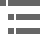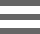## Refractive Index (Index of Refraction)Refractive Index (Index of Refraction) is a value calculated from the ratio of the speed of light in a vacuum to that in a second medium of greater density. The refractive index variable is most commonly symbolized by the letter n or n' in descriptive text and mathematical equations.

As presented in the figure above, a wavefront incident upon a plane surface separating two media is refracted upon entering the second medium if the incident wave is oblique to the surface. The incident angle (θ(1)) is related to the refraction angle (θ(2)) by the simple relationship known as Snell's law:

Where n represents the refractive indices of material 1 and material 2 and θ are the angles of light traveling through these materials with respect to the normal. There are several important points that can be drawn from this equation. When n(1) is greater than n(2), the angle of refraction is always larger than the angle of incidence. Alternatively when n(2) is greater than n(1) the angle of refraction is always smaller than the angle of incidence. When the two refractive indices are equal (n(1) = n(2)), then the light is passed through without refraction.

In optical microscopy, refractive index is an important variable in calculating numerical aperture, which is a measure of the light-gathering and resolving power of an objective. In most instances, the imaging medium for microscopy is air, but high-magnification objectives often employ oil or a similar liquid between the objective front lens and the specimen to improve resolution. The numerical aperture equation is given by:

where n is the refractive index of the imaging medium and θ is the angular aperture of the objective. It is obvious from the equation that increasing the refractive index by replacing the imaging medium from air (refractive index = 1.000) with a low-dispersion oil (refractive index = 1.515) dramatically increases the numerical aperture.

Snell's law was originally defined by the relationship between the incident angles and the ratio of the velocities of light in the two media. The refractive index or index of refraction is the ratio between the velocity of light (c) in free space (for all practical purposes, either air or a vacuum) and its velocity η in a particular medium:

As the refractive index of a material increases, the greater the extent to which a light beam is deflected (or refracted) upon entering or leaving the material. The refractive index of a medium is dependent (to some extent) upon the frequency of light passing through, with the highest frequencies having the highest values of n. For example, in ordinary glass the refractive index for violet light is about one percent greater than that for red light. A consequence of this phenomenon is that each wavelength experiences a slightly different degree of refraction when a heterogeneous light beam containing more than one frequency enters or leaves the medium. This effect is termed dispersion and is responsible for chromatic aberration in microscope objectives.

### Contributing Author

Michael W. Davidson - National High Magnetic Field Laboratory, 1800 East Paul Dirac Dr., The Florida State University, Tallahassee, Florida, 32310.

# Microscopy U - The source for microscopy education•••